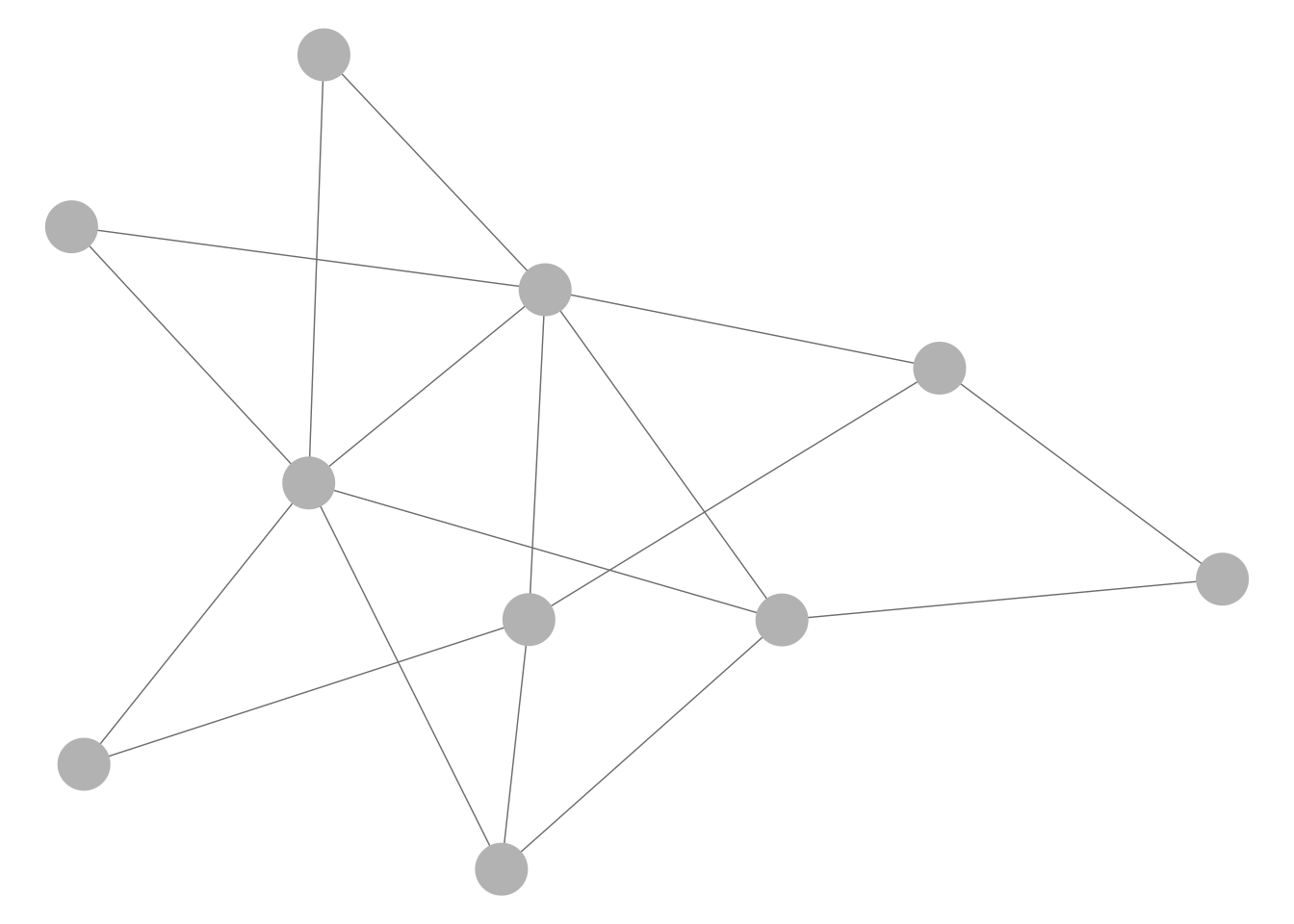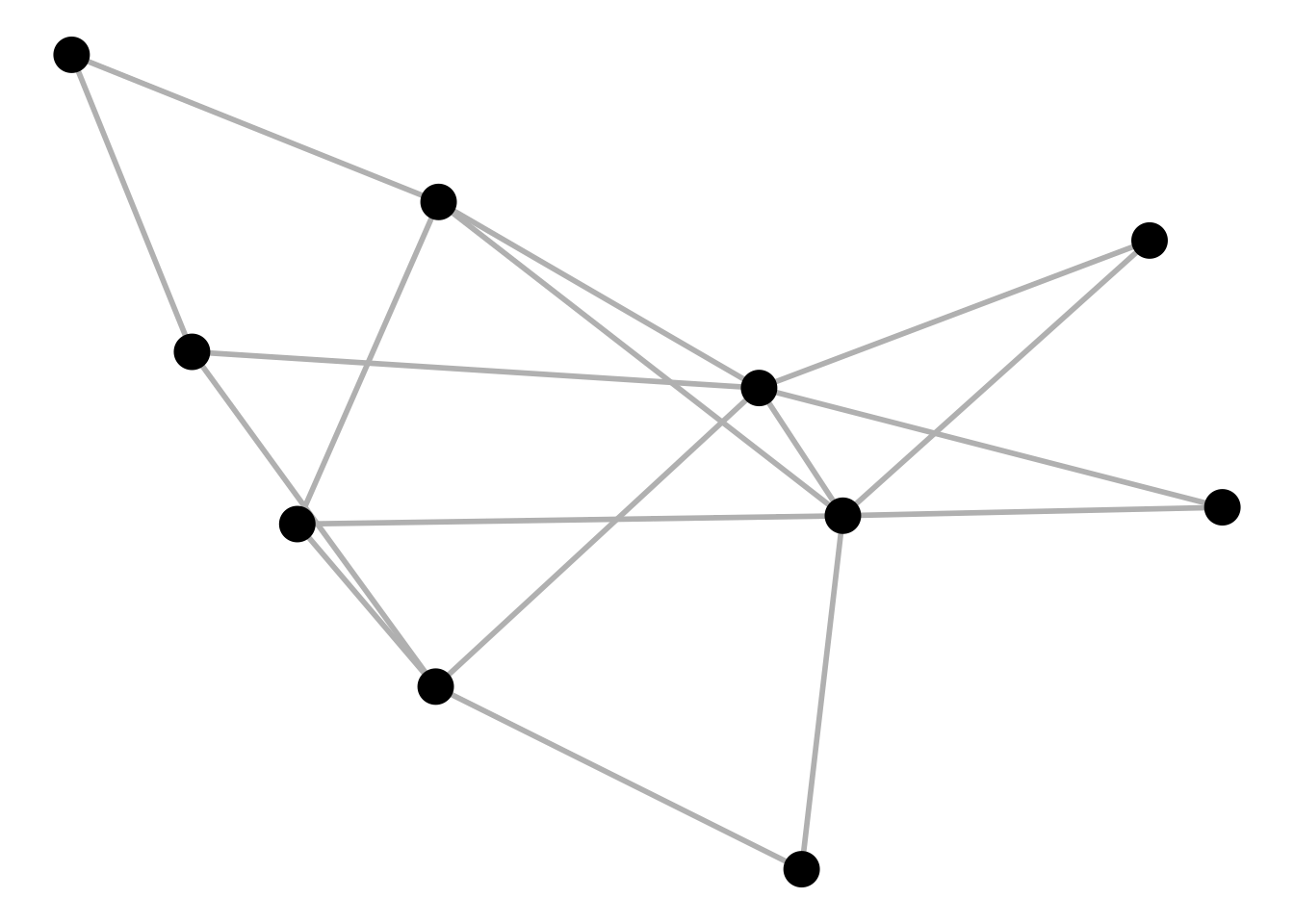# Introduction

The `ggnet2` function is a visualization function to plot network objects as `ggplot2` objects. It accepts any object that can be coerced to the `network` class, including adjacency or incidence matrices, edge lists, or one-mode `igraph` network objects.

## Rationale

`R` already provides many ways to plot static and dynamic networks, many of which are detailed in a beautiful tutorial by Katherine Ognyanova.

Furthermore, `R` can

All of these tools, however, require to use a new graph syntax, either within or outside of `R`, in order to create new network objects with the appropriate properties for plotting.

Instead, for the many users who are familiar with the `ggplot2` package, it might be interesting to use a syntax that comes close to its “grammar of graphics” to process and plot network data, in the same format as was used for network analysis.

This idea motivated the very first version of `ggnet`, by Moritz Marbach, and is also motivating the development of `geom_net`, a `geom` object for network data structured as data frames, by Sam Tyner and Heike Hofmann.

`ggnet2` is an improved version of `ggnet`. Both functions are available from the `GGally` package or as standalone functions. `ggnet2` brings several improvements that convey additional control over all plotting parameters.

## Installation

`ggnet2` is available through the `GGally` package:

``````install.packages("GGally")
library(GGally)``````

Or it can also be installed from its standalone package:

``````devtools::install_github("briatte/ggnet")
library(ggnet)``````

## Dependencies

The package dependencies of `ggnet2` are, on the one hand, the `network` and `sna` packages for network manipulation, and the `ggplot2` package for plot construction.

``````library(network)
library(sna)
library(ggplot2)``````

The `ggplot2` package will also load the `scales` package, which is used internally by `ggnet2`.

Additionally, `ggnet2` suggests the following packages:

All packages cited above can be installed from CRAN through `install.packages`.

## Notation

In this vignette, “nodes” designate the vertices of a network, and “edges” designate its ties. Readers who are not familiar with network terminology might want to consult a handbook such as Networks. An Introduction, by Mark Newman.

## Contents

Most of this vignette is organized around two simple network examples:

• node-level plotting parameters are demonstrated on a one-mode network, and
• edge-level plotting parameters are demonstrated on a two-mode (bipartite) network.

The vignette also contains a section that illustrates some additional capabilities of `ggnet2`, and another section showing two additional examples of real-world networks plotted with `ggnet2`. It closes on known limitations of `ggnet2`.

# Example (1): Random graph

Let’s start with an undirected Bernoulli random graph, with 10 nodes named “a, b, …, i, j”, and a rather high likelihood of an edge to exist between them:

``````# random graph
net = rgraph(10, mode = "graph", tprob = 0.5)
net = network(net, directed = FALSE)

# vertex names
network.vertex.names(net) = letters[1:10]``````

This graph can be visualized with `ggnet2` without any further work:

``ggnet2(net)``The `net` argument is the only compulsory argument of `ggnet2`. It can be a `network` object or any object that can be coerced to that class through its `edgeset.constructors` functions, such as adjacency matrixes, incidence matrixes and edge lists.

If the `intergraph` package is installed, `net` can also be an `igraph` one-mode network object, which is the only type of network that the package can convert from the `igraph` to the `network` class.

## Node color and size

The most basic properties that one might want to change at that stage are the size and color of the nodes, or the size and color of the edges. Let’s modify each of these properties:

``ggnet2(net, node.size = 6, node.color = "black", edge.size = 1, edge.color = "grey")``The vertex-related arguments of `ggnet2` start with `node`, and its edge-related arguments start with `edge`. The `node.color` and `node.size` arguments can be abbreviated:

``ggnet2(net, size = 6, color = "black", edge.size = 1, edge.color = "grey")``

It also possible to pass a vector of node colors directly to `ggnet2`, as long as it has the same number of elements as the network has nodes:

``ggnet2(net, size = 6, color = rep(c("tomato", "steelblue"), 5))``